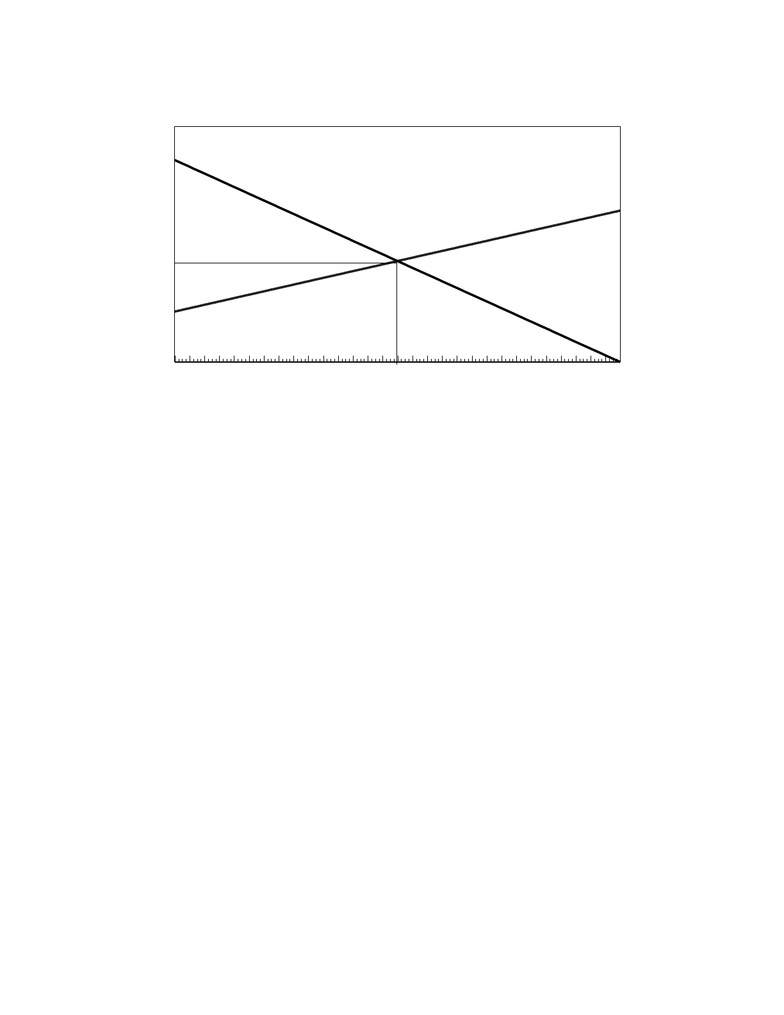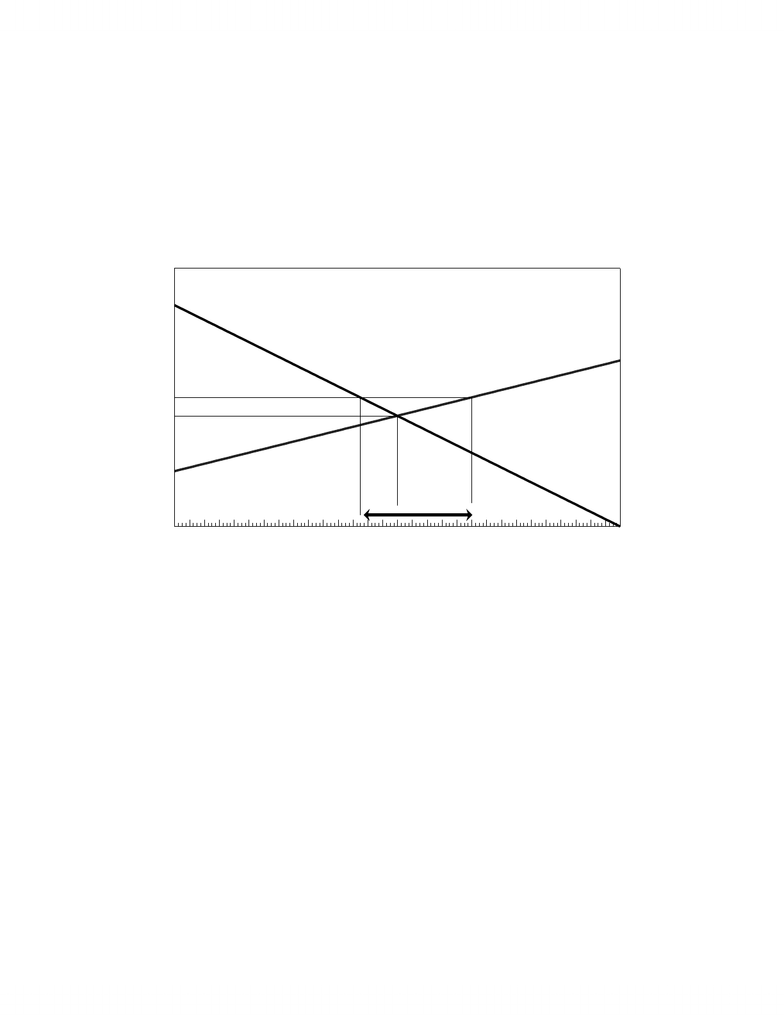# MGMT 1P93 Lecture Notes - Ceteris Paribus, Price Floor, Price Ceiling

23 views8 pagesWe can analyze Demand and Supply and Market equilibrium with linear equations. These
are equations of the form Y = a + bX where a is the Y-intercept, i.e., the value of Y when X is
zero, and b is the slope (rise/run = Y/X) of the function. We know that the function is linear,
i.e., a line, because the slope is constant. The slope is negative the Y falls with an increase in X
and is positive if Y increases with an increase in X.
Example: Suppose that the following equations describe the market Demand for and Supply of a
Economics book.
Demand: PD = 120 – 0.2QDSupply: PS = 30 + 0.1QS (P is in \$s and Q is in units)
We don’t need to label these equations Demand and Supply since the negative slope of the
first one and the positive slope of the second one tell us that they are Demand and Supply
respectively. Since equilibrium implies that PD = PS we simply equate the right-hand side of each
equation to find equilibrium.
Equilibrium => PD = PS => 120 – 0.2Q = 30 + 0.1Q
90 = 0.3Q => Q = 300
Q = 300 => P = 120 – 0.2*30 = \$60 or P = 30 + 0.2*30 = \$60
(P must be the same for Demand and Supply at equilibrium Q)
The following diagram depicts these equations and this equilibrium. Note that 120 is the
vertical (Y) intercept for Demand and that 30 is the vertical intercept for Supply. The horizontal
intercept for Demand is 120/0.2 = 600.
- 1 -
Unlock document

This preview shows pages 1-3 of the document.
Unlock all 8 pages and 3 million more documents.Demand for and Supply of an Economics Book
0
20
40
60
80
100
120
140
160
180
200
220
240
260
280
300
320
340
360
380
400
420
440
460
480
500
520
540
560
580
600
Quantity
0
20
40
60
80
100
120
140
Price (\$s)
Do
Supply
Demand
Po
Qo
GOVERNMENT IMPACT ON MARKETS
1. Fixed Prices
The government can fix prices to achieve policy objectives but there are economic
ramifications.
a) Price Floors (Minimum Prices)
A government may wish to protect producers against low prices by establishing a minimum
price (price floor) for a commodity. The classic commodities for price floors have been
agricultural products such as eggs, milk, or peanuts, but minimum wages and minimum
exchange rates are also common historically. A price floor is effective only if it is above the
equilibrium price since the market would move to the equilibrium price.
Governments assume or hope that the minimum price is a temporary measure to help
producers in a depressed market but the existence of a price floor above the equilibrium price at
that point results in surplus (unsold) commodities. It is difficult to simply decree minimum
prices since some producers will sell below minimum price on the black market thereby driving
- 2 -
Unlock document

This preview shows pages 1-3 of the document.
Unlock all 8 pages and 3 million more documents.down the price. Governments usually have to establish the price floor, therefore, by purchasing
surplus commodities.
Example #1. Suppose that the government enacts a minimum price on textbooks by promising to
buy any surplus commodities at \$70. The following diagram depicts this situation.
Goverment Price Floor
0
20
40
60
80
100
120
140
160
180
200
220
240
260
280
300
320
340
360
380
400
420
440
460
480
500
520
540
560
580
600
0
20
40
60
80
100
120
140
Price (\$s)
Supply
Demand
Po
P
Floor
Qs
Qd
Surplus
We can find the specific amount of the surplus and the cost to the government of the price
floor by calculating the surplus as the difference between the quantity demanded and the quantity
supplied in the market at \$70
\$70 = 120 – 0.2QD=> Qd = (120 – 70)/0.2 = 250
\$70 = 30 + 0.1QS => Qs = (70 – 30)/0.1 = 400
=> Surplus = Qd – Qs = 400 – 250 = 150
Cost to the Government in buying this surplus = 150*70 = \$10,500
Total Revenue of Firms = 400 * 70 = \$28,000
Total Expenditure of Consumers = 250*70 = \$17,500
NOTE: Price floors supported by government purchases have two problems:
- 3 -
Unlock document

This preview shows pages 1-3 of the document.
Unlock all 8 pages and 3 million more documents.

# Get access

\$10 USD/m
Billed \$120 USD annually
Homework Help
Class Notes
Textbook Notes